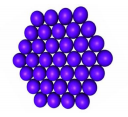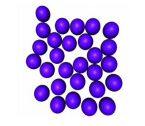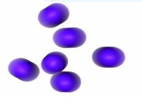### Matter in Our Surrounding - Revision Notes

CBSE Class 9 Science

Revision Notes
CHAPTER – 1
Matter in our Surroundings

• Anything that occupies space and has mass and is felt by senses is called matter.
• According to indian ancient philosphor, matter is the form of five basic elements (the Panchtatva) – air , earth ,fire , sky and water.

Characteristics of particles of matter

• Vacant spaces exist between particles.
• Particles are in continuous motion.
• Particles are held together by forces of attraction.

States of Matter
Basis of Classification of Types

• Based upon particle arrangement
• Based upon energy of particles
• Based upon distance between particles

Five states of matter

1. Solid
2. Liquid
3. Gas
4. Plasma
5. Bose-Einstein condensate

(I) SOLID• Fixed mass, volume and shape
• Inter-particle distances are least.
• Incompressible.
• High density and do not diffuse
• Inter particle forces of attraction are strongest.
• Constituent particles are very closely packed.

(II) LIQUID• Not fixed shape but fixed volume and mass.
• Inter particle distances are larger than solid.
• Almost incompressible.
• Density is lower than solids and can diffuse.
• Inter particle forces of attraction are weaker than solids .
• Constituent particles are less closely packed.

(III) GAS• Neither fixed shape nor fixed volume.
• Inter particle distances are largest.
• Highly compressible.
• Density is least and diffuse.
• Inter particle forces of attraction are weakest.
• Constituent particles are free to move about.

(IV) PLASMA (NON-EVALUATIVE)

• A plasma is an ionized gas.
• A plasma is a very good conductor of electricity and is affected by magnetic fields.
• Plasma, like gases have an indefinite shape and an indefinite volume. Ex. Ionized gas

(v) BOSE-EINSTEIN CONDENSATE (non –evaluative)

• BEC is a state of matter that can arise at very low temperatures.
• The scientists who worked with the Bose-Einstein condensate received a Nobel Prize for their work in 1995.
• The BEC is all about molecules that are really close to each other (even closer than atoms in a solid).

Microscopic Explanation for Properties of Solids

• Solids have a definite shape and a definite volume because the particles are locked into place
• Solids do not flow easily because the particles cannot move/slide past one another
• Solids are not easily compressible because there is little free space between particles

Microscopic Explanation for Properties of Liquids

• Liquids are not easily compressible and have a definite volume because there is little free space between particles.
• Liquids flow easily because the particles can move/slide past one another.
• Liquids flow easily because the particles can move/slide past one another.

Microscopic Explanation for Properties of Gases

• Gases are easily compressible because there is a great deal of free space between particles
• Gases flow very easily because the particles randomly move past one another.
• Gases have an indefinite shape and an indefinite volume because the particles can move past one another (non –evaluative)

Microscopic Explanation for Properties of Plasmas

• Plasmas have an indefinite shape and an indefinite volume because the particles can move past one another.
• Plasmas are easily compressible because there is a great deal of free space between particles.
• Plasmas are good conductors of electricity &are affected by magnetic fields because they are composed of lens.

Microscopic Explanation for Properties of BEC

• Particles are less energetic than solids because Exist at very low temperature.
• Particles are literally indistinguishable because they are locked into same space .
• BEC shows super fluidity because Particles can flow without friction.
1. Interchange in states of matter

Water can exist in three states of matter –

• Solid, as ice,
• Liquid, as the familiar water, and
• Gas, as water vapour.

Sublimation : The changing of solid directly into vapours on heating & vapours into solid on cooling. Ex. Ammonium chloride, camphor & iodine.

(a) Effect of change in temperature

The temperature effect on heating a solid varies depending on the nature of the solid & the conditions required in bringing the change.

• On increasing the temperature of solids, the kinetic energy of the particles increases which overcomes the forces of attraction between the particles thereby solid melts and is converted to a liquid.
• The temperature at which a solid melts to become a liquid at the atmospheric pressure is called its melting point.
• The melting point of ice is 273.16 K.
• The process of melting, that is, change of solid state into liquid state is also known as fusion.

(b) Effect of Change of Pressure

• Increasing or decreasing the pressure can change the state of matter. Applying pressure and reducing temperature can liquefy gases.
• Solid carbon dioxide ($C{O}_{2}$) is stored under high pressure. Solid $C{O}_{2}$ gets converted directly to gaseous state on decrease of pressure to 1 atmosphere without coming into liquid state. This is the reason that solid carbon dioxide is also known as dry ice.

Latent Heat :

The hidden heat which breaks the force of attraction between the molecules during change of state.

Fusion Heat energy required to change 1kg of solid into liquid.

Vaporisation Heat energy required to change 1kg of liquid to gas at atmospheric pressure at its boiling point

Thus, we can say that pressure and temperature determine the state of a substance, whether it will be solid, liquid or gas.

4. Evaporation & Boiling

• Particles of matter are always moving and are never at rest.
• At a given temperature in any gas, liquid or solid, there are particles with different amounts of kinetic energy.
• In the case of liquids, a small fraction of particles at the surface, having higher kinetic energy, is able to break away from the forces of attraction of other particles and gets converted into vapour .
• This phenomenon of change of a liquid into vapours at any temperature below its boiling point is called evaporation.

Factors Affecting Evaporation

• The rate of evaporation increases with an increase of surface area.
• With the increase of temperature, more number of particles get enough kinetic energy to go into the vapour state.
• Humidity is the amount of water vapour present in air. The air around us cannot hold more than a definite amount of water vapour at a given temperature. If the amount of water in air is already high, the rate of evaporation decreases.
• Wind speed : the higher the wind speed , the more evaporation.

Evaporation cause cooling.

• The particles of liquid absorb energy from the surrounding to regain the energy lost during evaporation,

Evaporation Vs Boiling

• Boiling is a bulk phenomenon. Particles from the bulk (whole) of the liquid change into vapour state.
• Evaporation is a surface phenomenon. Particles from the surface gain enough energy to overcome the forces of attraction present in the liquid and change into the vapour state.

5. Kelvin & Celsius Scale

• Kelvin is the SI unit of temperature, ${0}^{\circ }C$ = 273.16 K we take ${0}^{\circ }C$ = 273 K.
• SI unit of temperature is Kelvin. $T\phantom{\rule{thickmathspace}{0ex}}\left(K\right)=T{\left(}^{0}C\right)+273$
• Kelvin scale of temperature has always positive sign , hence regarded as better scale than Celsius.
• Atmosphere (atm) is a unit of measuring pressure exerted by a gas. The SI unit of pressure is Pascal (Pa):
• 1 atmosphere = 1.01 × (10 to the power 5) Pa. The pressure of air in atmosphere is called atmospheric pressure. The atmospheric pressure at sea level is 1 atmosphere, and is taken as the normal atmospheric pressure.

You are expected to know

• Particle nature of matter.
• All five states of matter & their behaviour
• enter conversion of states of matter
• Latent heat
• Conversion between Kelvin scale & Celsius scale